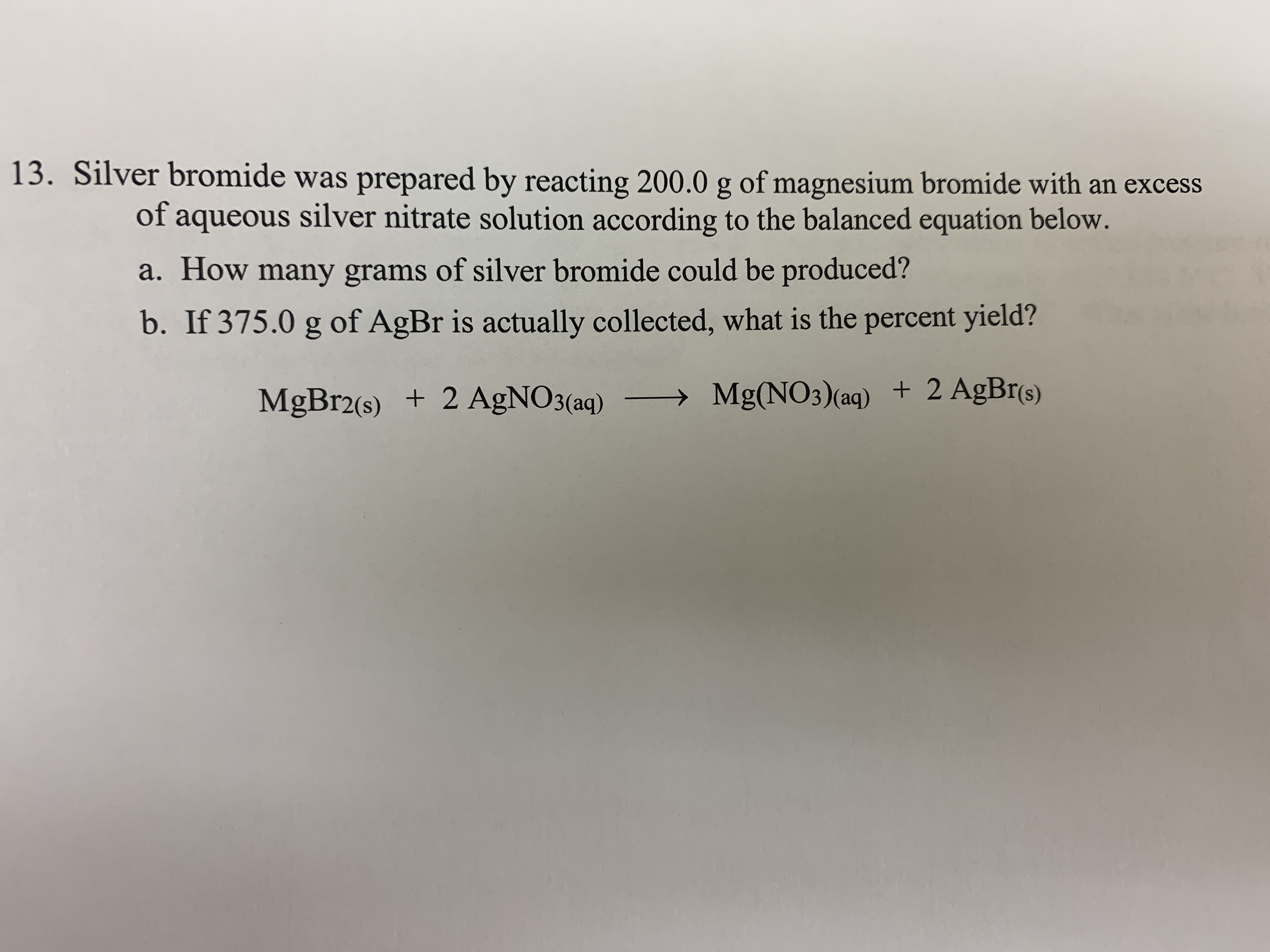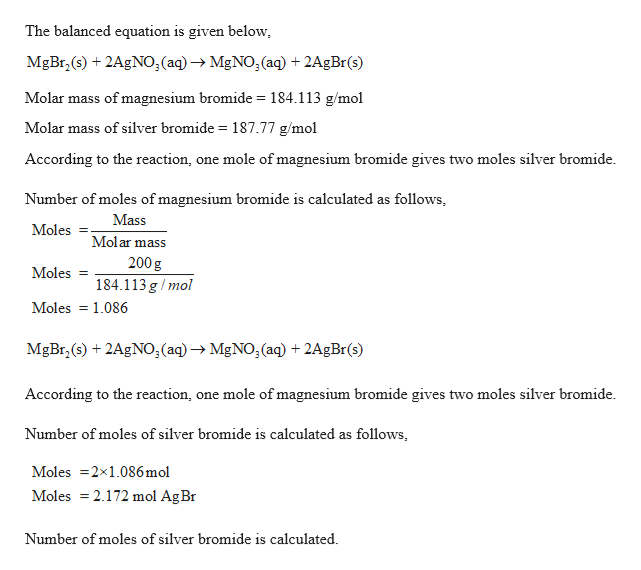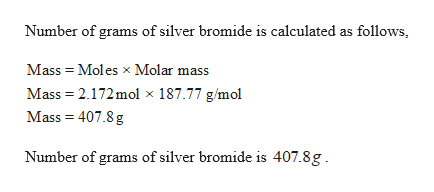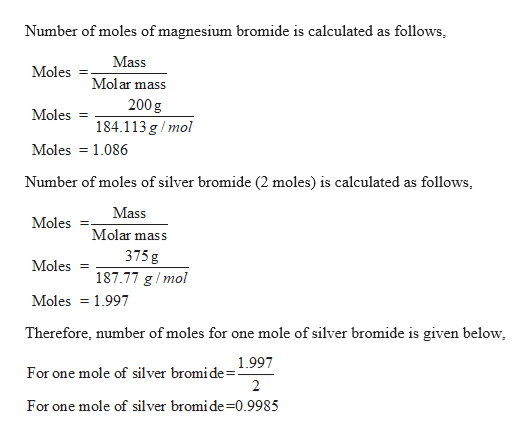# 13. Silver bromide was prepared by reacting 200.0 g of magnesium bromide with an excessof aqueous silver nitrate solution according to the balanced equation below.a. How many grams of silver bromide could be produced?b. If 375.0 g of AgBr is actually collected, what is the percent yield?MgBr2(s) 2 AgNO3(aq)>Mg(NO3)(aq) 2 AgBr(s)

Question
108 viewshelp_outlineImage Transcriptionclose13. Silver bromide was prepared by reacting 200.0 g of magnesium bromide with an excess of aqueous silver nitrate solution according to the balanced equation below. a. How many grams of silver bromide could be produced? b. If 375.0 g of AgBr is actually collected, what is the percent yield? MgBr2(s) 2 AgNO3(aq) >Mg(NO3)(aq) 2 AgBr(s) fullscreen
check_circle

Step 1

Given,

200.0 g of magnesium bromide.help_outlineImage TranscriptioncloseThe balanced equation is given below MgBr (s)2AgNO,(aq)- MgNO,(aq) +2AgBrs) Molar mass of magnesium bromide = 184.113 g/mol Molar mass of silver bromide = 187.77 g/mol According to the reaction, one mole of magnesium bromide gives two moles silver bromide. Number of moles of magnesium bromide is calculated as follows, Mass Moles Molar mass 200g Moles 184.113g/mol Moles 1.086 MgBr(s)2AgNO3(aq)-MgNO2(aq) + 2AgBr(s) According to the reaction, one mole of magnesium bromide gives two moles silver bromide. Number of moles of silver bromide is calculated as follows Moles 2x1.086 mol Moles 2.172 mol Ag Br Number of moles of silver bromide is calculated fullscreen
Step 2

Number of grams of silver bromide is calculated.help_outlineImage TranscriptioncloseNumber of grams of silver bromide is calculated as follows Mass Moles x Molar mass Mass 2.172mol x 187.77 g/mol Mass 407.8 g Number of grams of silver bromide is 407.8g fullscreen
Step 3

Percent yield has to ...help_outlineImage TranscriptioncloseNumber of moles of magnesium bromide is calculated as follows Mass Moles Molar mass 200 g Moles 184.113 g/mol Moles 1.086 Number of moles of silver bromide (2 moles) is calculated as follows Mass Moles Molar mass 375 g Moles 187.77 g/mol Moles 1.997 Therefore, number of moles for one mole of silver bromide is given below 1.997 For one mole of silver bromi de= 2 For one mole of silver bromide-0.9985 fullscreen

### Want to see the full answer?

See Solution

#### Want to see this answer and more?

Solutions are written by subject experts who are available 24/7. Questions are typically answered within 1 hour.*

See Solution
*Response times may vary by subject and question.
Tagged in

### General Chemistry